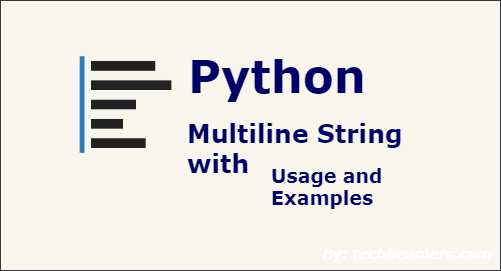# How to Create Multiline String in Python

Python allows you to create multiline strings to accommodate large strings. But there is a specific way to define multiline strings in python. You cannot wrap it in single or double quotes like you do for single line strings. In this article, we will learn how to create multiline strings in Python. In fact, multiline strings also allow you to pass variables in them. We will also learn how to do this.

## How to Create Multiline String in Python

There are two ways to define a multiline strings – by wrapping it in 3 double quotes, or 3 single quotes, as shown below.

```a = """Lorem ipsum dolor sit amet,
sed do eiusmod tempor incididunt
ut labore et dolore magna aliqua."""
print(a)```

OR

```a = '''Lorem ipsum dolor sit amet,
sed do eiusmod tempor incididunt
ut labore et dolore magna aliqua.'''
print(a)```

## Add Inline Variables in Multiline strings

You can also use format() function to add inline variables in your single or multiline strings, as shown below.

```>>> s = "This is an {example} with {vars}".format(vars="variables", example="example")
>>> s
'This is an example with variables'```

In the above code, we specify 2 variables example and vars in a multiline string ‘s’. We use format function to define both these variables. Inside format function, you can define all the variables that you mentioned in your single line string.

Similarly, you can use format() function to define variables in multiline strings.

```>>> s = '''\
... This is a {first} example.
... Here is a {second} line.\
... '''.format(first='multi-line', second='second')
>>> print(s)
This is a multi-line example.
Here is a second line.```

In the above example, we define two variables first and second and define them in format() function.

You can also use a dictionary of key-value pairs for inline variables in your string. The keys act as variables and their values act as variable values. Here is an example.

```>>> d = { 'vars': "variables", 'example': "example" }
>>> s = "This is an {example} with {vars}"
>>> s.format(**d)
'This is an example with variables'```

In the above code, we have defined d dictionary with a couple of variables vars and example. We have used format() function to input these variables in our multiline string. In this case, it is important to note that you need to provide the reference to the dictionary (**d) and not the dictionary itself, in the format() function.

Alternatively, you can also use format specifiers in your string, as shown below.

```string1 = "go"
string2 = "now"

s = """
I will %s there
I will go %s
""" % (string1, string2)

print(s)```

In the above example, we use %s to specify placeholders for string variables which we specify using % (string1, string2). But in this case, the order of variables is important since the first %s in our string is substituted with string1, the second %s is substituted with string2 and so on. So it is advisable to use format() function.

In this article, we have learnt many different ways to define multiline strings in Python, and also use inline variables in it.